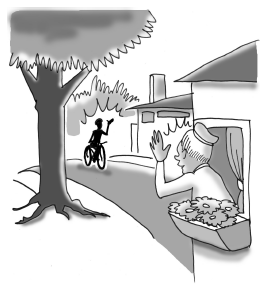### Home > AC > Chapter 11 > Lesson 11.1.2 > Problem11-24

11-24.Ten minutes after he left his home, Gerald was $40$ miles from his grandmother’s house. Then, $22$ minutes after he left, he was $34$ miles from her house. If he was traveling toward his grandmother’s home at a constant rate and reached her house after $90$ minutes, how far away from her house does he live?

Write two equations for the distance and solve like a system of equations.

$d=\text{distance}$
$r=\text{rate}$

$d=10r+40$
$d=22r+34$
Use the equal values method.

$d = 45 \; \text{miles}$